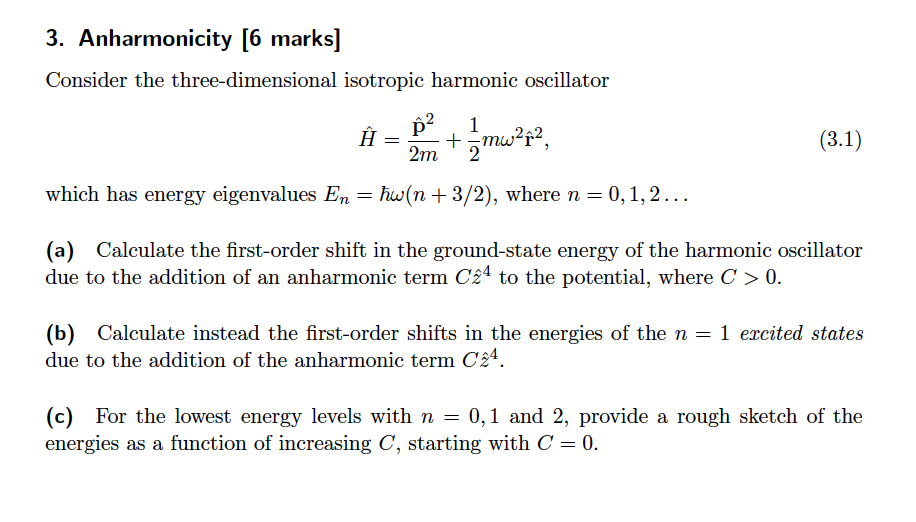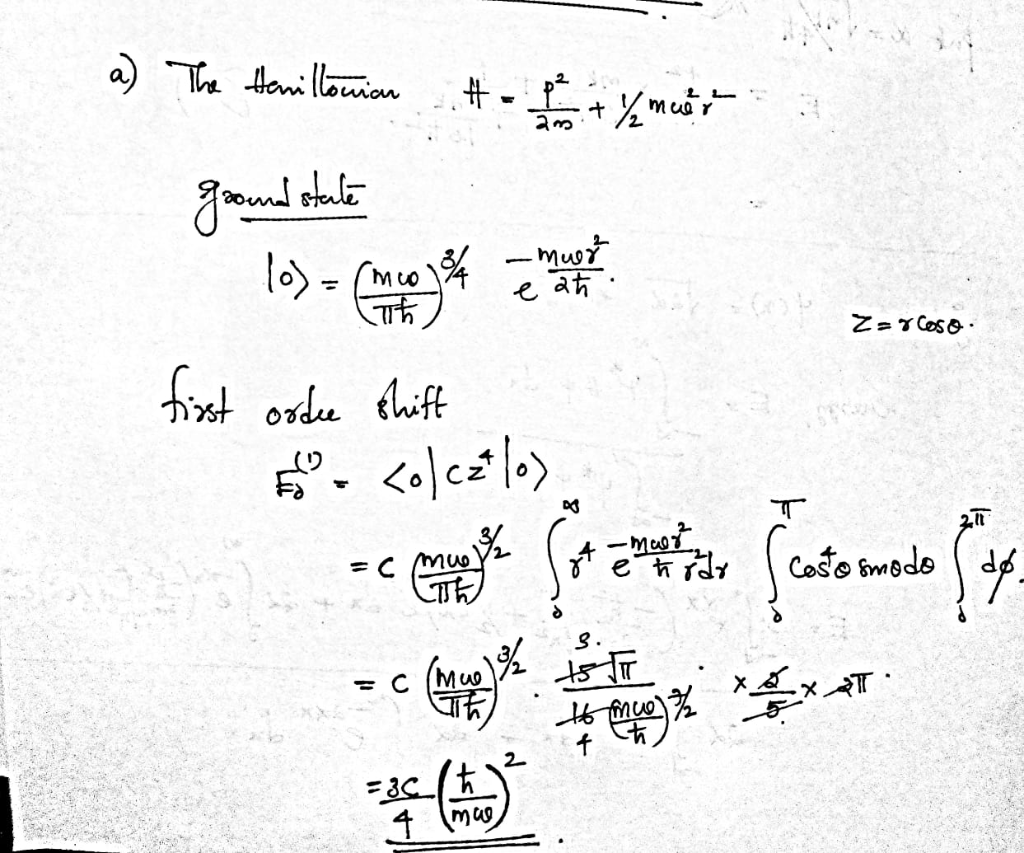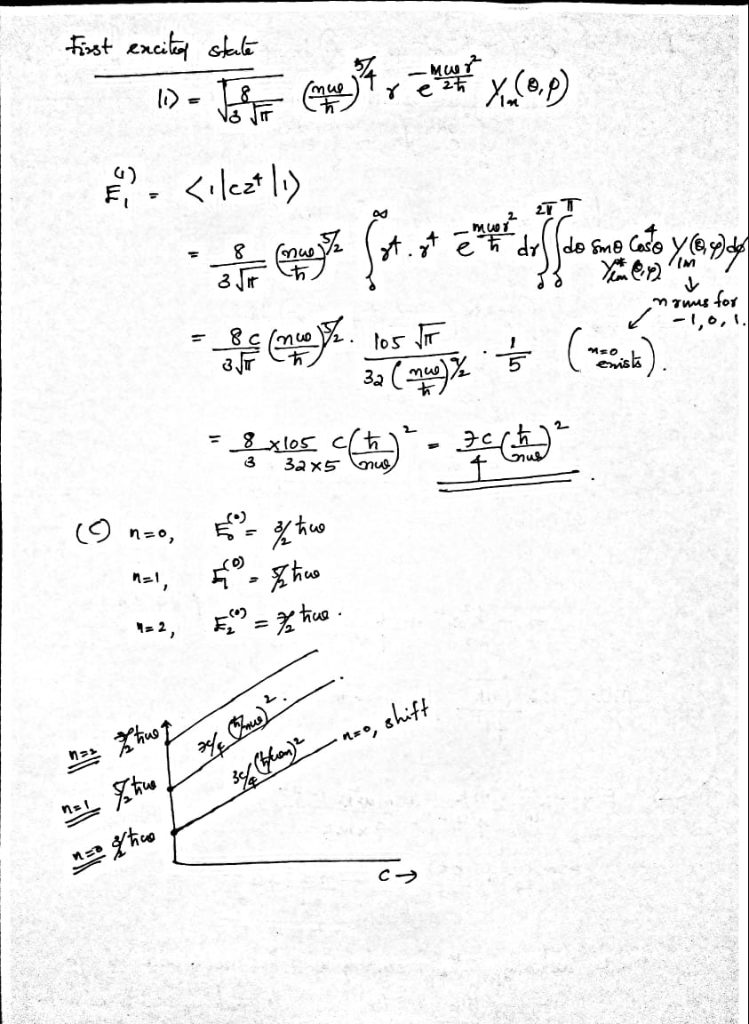# 3. Anharmonicity (6 marks] Consider the three-dimensional isotropic harmonic oscillator 2 1 242 р...3. Anharmonicity (6 marks] Consider the three-dimensional isotropic harmonic oscillator 2 1 242 рґ which has energy eigenvalues En-hu(n+3/2), where n- 0,1,2.. (a) Calculate the first-order shift in the ground-state energy of the harmonic oscillator due to the addition of an anharmonic term C24 to the potential, where C> 0. (b) Calculate instead the first-order shifts in the energies of the n - 1 ercited states due to the addition of the anharmonic term C (c) For the lowest energy levels with n -0,1 and 2, provide a rough sketch of the energies as a function of increasing C, starting with C 0.##### Add Answer of: 3. Anharmonicity (6 marks] Consider the three-dimensional isotropic harmonic oscillator 2 1 242 р...
More Homework Help Questions Additional questions in this topic.